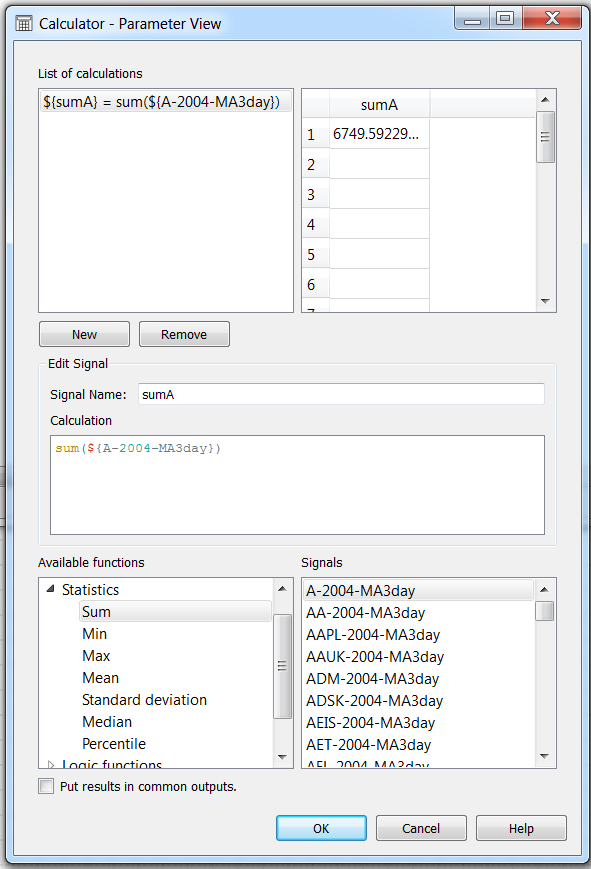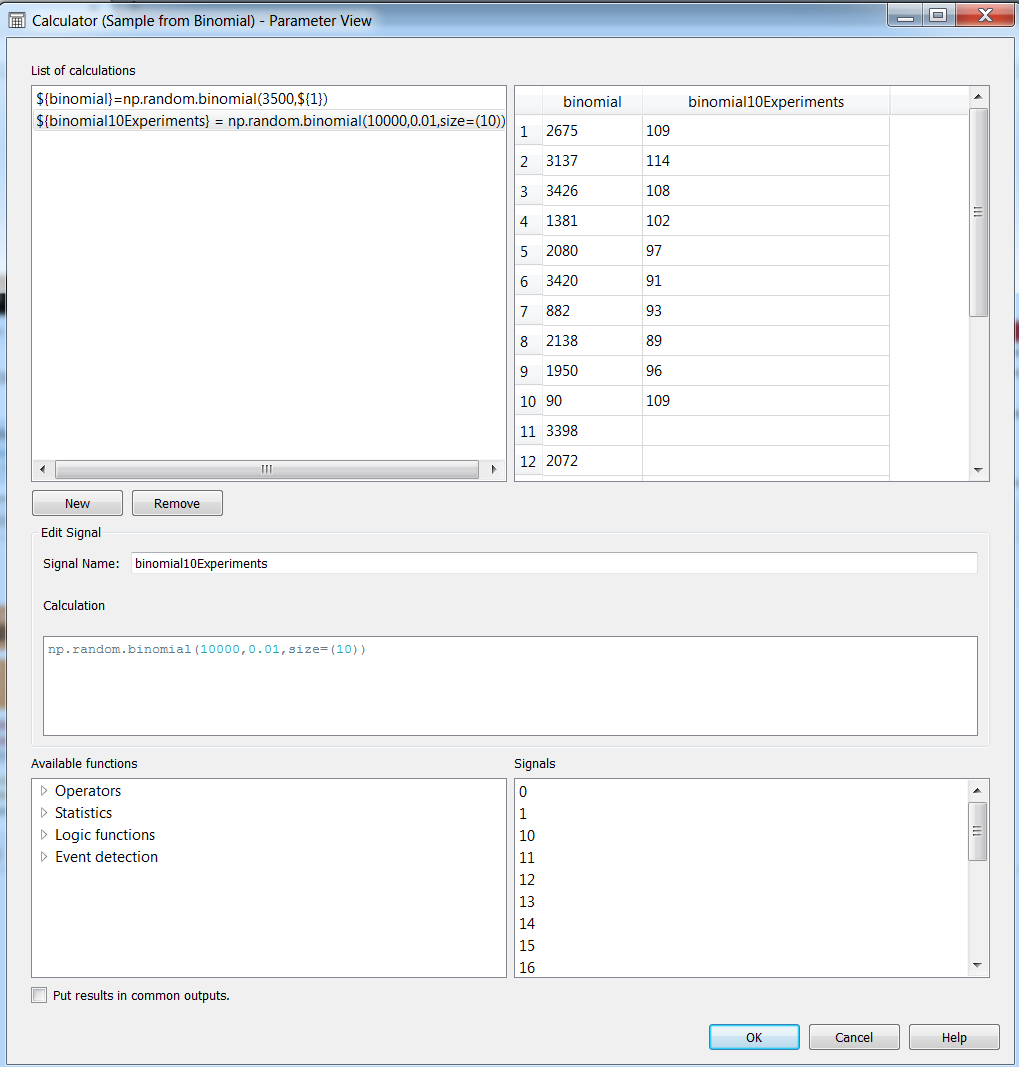Basic Statistics with Sympathy – Part 2: Plotting and using the Calculator Node for common functions. | Combine

Allow me to introduce you to your new best friend from Sympathy 1.2.x: The improved calculator node. The node takes a list of tables, from which you can establish a new signal with the output for a calculation. There is already a menu with the most popular calculations and a list of signals from the input tables, all in the same configuration window.

To do this tutorial, it is recommended to use some data in csv format, and the latest version of Sympathy for Data (1.2.5 at the time of writing this post).If you would like to more complex formats (say, INCA-files) here are some pointers that can help you on that.

Now, with your data set and ready, follow the steps in here and locate the nodes to build the following flow:

And then right-click on the “Calculator” node to open the configuration window for the node. Now, lets get our first calculation going!

Our First Calculation: A Column operation

1. Write a Signal Name (you don’t have to do it as the first step, but it helps you keep track of your work). In this example, we have chosen to write “sumA” since we intend to obtain the sum of the values of a signal whose name starts with A.
2. Drag-and-drop a function from “Available functions” into “Calculation”. In this example, we have chosen to drag “Sum”.
3. Drag-and-drop a signal name into approximately the point in the calculation in which the variable name is supposed to go.

You may need to delete some remaining text in the calculation until the preview in the top right shows you a correct number. It should look similar to the following screen:First calculation. Note that the preview in the top right shows you a valid value

Second Calculation: A Row-wise operation

Now lets create a new calculation: click on “New” (on top of “Edit Signal”). We will now make a new signal that will be the sum of two signals, elementwise. As you can see in the figure below, this signal will be called “sumTwoSignals”. So, simply drag-and-drop the names of the signals you want to sum, and make sure you put the operator between the two names. In this case, the operator was a sum (+).

What happens if the calculation outputs differ in shape?

In many cases (like in this example), the calculations may not have the same dimensions, and you may want to separate them into different output tables, because then Sympathy will inform you that it can not put together two columns with different lengths on the same table. For those cases, uncheck the “Put results in common outputs” box, and then the results will go to different columns. In newer versions of sympathy the checkbox “Put results in common output” is checked by default.

Now, lets go into something more interesting…

You can use the principles of the previous two sections together with the operators in “available functions” in order to accomplish many day-to-day tasks involving calculations over your data.

But there is more fun from where all of that came from!. The real hidden superpower of your new best friend relies on the fact that you can access numpy from it. Yes, you can have the best of both worlds: the power of numpy from a graphic interface! Your colleague can stay in French Guyana and watch some space launches, you have almost everything you can ever dream of!

For our next example, lets build a binomial distribution. All you need to do is to write in the calculation field:

np.random.binomial(number_of_tests,success_pr,size=(x))

In which “x” is the number of instances for the trials. This will give you a signal of size “x”, from which each element in the output signal is the number of successful outcomes out of “number_of_tests” for an event with probability “success_pr” of succeeding. You can now manually assign a value to those elements. Notice in the figure below that you can either hand-type a set of values in there for quick estimations, or use variable names from input signals as inputs to the function.Examples for using np.random.binomial with hand-written parameters and with signals from input files

Building and plotting a PDF (Probability Density Function)

A nice feature of the histograms in numpy, is that you can use them to build probability density functions by setting the parameter density to True (see numpy documentation entry here). Since np.histogram will return two arrays (one with the PDF, and one with the bin_edges) then you may want to also add a  in the end. So, it can look like this:

np.histogram(${yourVariable},bins=10,density=True) Now lets plot the distribution!. To be able to plot anything, since the plotting in sympathy is based upon matplotlib, you will require an independent axis, form which your PDF will be the dependent axis. You could take the other returning value of the histogram for that purpose, by writing a new calculation which will serve as the new independent axis for plotting: np.histogram(${NVDA-2006-MA3day},bins=10,density=False)[:-1]

Note that the length of the bin edges are always length(hist)+1, and here we have chosen the left as the start of the distribution.  A simple way to be clean about what you intend to plot, is to extract the signals and then hjoin then in a table, which will be fed to the “Plot Table” module in sympathy. It can look like this:

In the configuration menu of the “Plot Table” module, you can select the labels for the independent (X) and dependent (Y) axis, the scale, ticks and if you would like to add a grid into the plot. In the “Signal” Tab, you can select which signal goes to which axis, and the colours and style of the plot. See below an example of a plot with 10 bins, a plot with 100 bins, and a plot with 1000 bins.

We hope that with this tutorial you can have some tools to study your data. And if you get bored and start daydreaming about your colleague at French Guyana, you can always watch this video. Have fun!